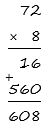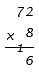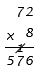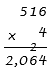# Multi-Digit Multiplication

## Objective

Multiply four-digit, three-digit, and two-digit numbers by one-digit numbers and assess the reasonableness of the product.

## Common Core Standards

### Core Standards

?

• 4.NBT.B.5 — Multiply a whole number of up to four digits by a one-digit whole number, and multiply two two-digit numbers, using strategies based on place value and the properties of operations. Illustrate and explain the calculation by using equations, rectangular arrays, and/or area models.

?

• 3.NBT.A.3

• 4.NBT.A.1

• 4.NBT.B.4

• 3.OA.B.5

• 3.OA.C.7

## Criteria for Success

?

1. Multiply a two-, three-, or four-digit whole number by a one-digit whole number using the partial products algorithm and/or the standard algorithm.
2. Assess the reasonableness of answers using mental computation and estimation strategies including rounding (MP.1).
3. Solve one-step word problems involving multiplication of up to four-digit by one-digit numbers (on the Problem Set and Homework) (MP.4).

## Tips for Teachers

?

Note that the last Criteria for Success is not addressed in the tasks below, since each problem type involving multiplication has been explored in previous lessons. However, if it seems students need additional practice with any type (particularly multiplicative compare with larger unknown), then you can use the values in Anchor Tasks #2 or #3 in the context of a word problem.

#### Remote Learning Guidance

If you need to adapt or shorten this lesson for remote learning, we suggest prioritizing Anchor Task 1 (benefits from worked example) and Anchor Task 2 (can be done independently). Find more guidance on adapting our math curriculum for remote learning here.

#### Fishtank Plus

• Problem Set
• Student Handout Editor
• Vocabulary Package

?

### Problem 1

Patrice solved the problem 72 x 8 using the partial products algorithm, as shown below:Jerome solved in a different way from Patrice. Here is what he did:

 First, he found the partial product of 2 and 8, which is 16. He wrote the ones digit, 6, under the equal bar, and the 1 ten on the equal bar above the tens place in smaller handwriting.Then, he found the partial product of 7 tens and 8, which was 56 tens. Because he had the 1 ten from the first partial product, he added those tens together and wrote 57 in the hundreds and tens place in the product. Because he had accounted for the 1 ten from his first partial product, he crossed it off.1. How is Jerome’s method similar to and different from Patrice’s method?
2. What are the advantages and disadvantages of Jerome’s method? Of Patrice’s method?

### Problem 2

Jerome’s method is called the standard algorithm. Use this method to find the following products. Then assess the reasonableness of your answer.

a. 54 x 6

b. 9 x 673

c. 1,605 x 4

## Problem Set & Homework

#### Discussion of Problem Set

• Look at #1. Which problems were particularly difficult to solve? Why?
• Look at #2. Were there any answers you could eliminate right away as being unreasonable?
• Look at #4. Where did the 3 come from? What value does it have—ones? Tens? Hundreds? Thousands? What is the final product?
• Look at #5. What is a possible number that Casey multiplies 178 by? Are there other possibilities? How did you find them? What product do they result in?
• Did you use any strategies from Lesson 11 to solve any problems on today’s Problem Set? For example, #1d?

?

### Problem 1

A student solved 516 x 4 below. There is a 2 above the tens digit in the product. What does that 2 represent?### Problem 2

a.      61 x 5                                b.      6 x 2,348

### Mastery Response

?